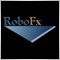# Can somebody help to code indicator?225

Hi, Can somebody help to code indicator?

Upper Band=(1+M1/100) x MA(close,N)
Lower Band=(1-M2/100) x MA(close,N)
Middle band=( Upper Band+Lower Band )/2

M1 & M2---->6
N--->25

Thank.1167

WWMMACAU:
Hi, Can somebody help to code indicator?

Upper Band=(1+M1/100) x MA(close,N)
Lower Band=(1-M2/100) x MA(close,N)
Middle band=( Upper Band+Lower Band )/2

M1 & M2---->6
N--->25

Thank.

What is N? Is it the period of MA or shift?
Also what is the point of the middle line? It will always be equal to MA.225

robofx.org:

What is N? Is it the period of MA or shift?
Also what is the point of the middle line? It will always be equal to MA.

N is period, as 25 days.

Thanks.1167

WWMMACAU:

N is period, as 25 days.

Thanks.

Here it is.

Files:225

robofx.org:

Here it is.

Thanks a lot, robofx.org.225

robofx.org:

Here it is.

Hi robofx.org.

Middle band(Magenta) only is appeared (drawn) when I attached BandsIndi.mq4 to my chart.

Thanks19976

Middle band(Magenta) only is appeared (drawn) when I attached BandsIndi.mq4 to my chart.
Huh? Output of an indicator can only appear if attached to a chart.41

WWMMACAU wrote >>

Hi robofx.org.

Middle band(Magenta) only is appeared (drawn) when I attached BandsIndi.mq4 to my chart.

Thanks

you only see middle line, because the outbands are far away from middle line
use smaller M1 and M2, you will see the other bands

AM1167

WWMMACAU:

Hi robofx.org.

Middle band(Magenta) only is appeared (drawn) when I attached BandsIndi.mq4 to my chart.

Thanks

All three lines are there, but if you are looking at a short period chart you'll see only the middle line (which is MA).
Switch to D1 and you will see the upper and lower bands.225

robofx.org:

All three lines are there, but if you are looking at a short period chart you'll see only the middle line (which is MA).
Switch to D1 and you will see the upper and lower bands.

Thanks, robofx.org. i got it now.10

lot increase by every new win i want it to repeat every 25 win but in this code it only do the first 25 win and then stop
i want every 25 win it start again from the beginning and keep repeating that

double LotsOptimized()
{
double lot=0.1;
int orders=HistoryTotal(); // history orders total
int losses=0,win=0; // number of losses orders without a break
//---- select lot size
//---- calcuulate number of losses orders without a break
if(DecreaseFactor>0)
{
for(int i=orders-1;i>=0;i--)
{
if(OrderSelect(i,SELECT_BY_POS,MODE_HISTORY)==false) { Print("Error in history!"); break; }
if(OrderSymbol()!=Symbol() || OrderType()>OP_SELL) continue;
//----
if(OrderProfit()>0) win++;
if(OrderProfit()<0) losses++;
}
}
//---- return lot size
if(win==1) lot=0.2;
if(win==2) lot=0.3;
if(win==3) lot=0.4;
if(win==4) lot=0.5;
if(win==5) lot=0.6;
if(win==6) lot=0.7;
if(win==7) lot=0.8;
if(win==8) lot=0.9;
if(win==9) lot=1.0;
if(win==10) lot=1.1;
if(win==11) lot=1.2;
if(win==12) lot=1.3;
if(win==13) lot=1.4;
if(win==14) lot=1.5;
if(win==15) lot=1.6;
if(win==16) lot=1.7;
if(win==17) lot=1.8;
if(win==18) lot=1.9;
if(win==19) lot=2.0;
if(win==20) lot=2.1;
if(win==21) lot=2.2;
if(win==22) lot=2.3;
if(win==23) lot=2.4;
if(win==24) lot=2.5;
return(lot);
}